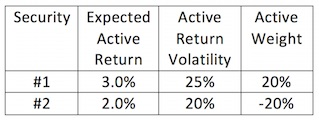### CFA Practice Question

There are 255 practice questions for this topic.

### CFA Practice Question

Consider two individual securities whose active returns are independent.Suppose the benchmark portfolio for the two securities is equally weighted (50% for each security) and the forecasted return on the benchmark portfolio is 5%. According to the basic fundamental law, the information coefficient is ______.

Portfolio weights and total expected returns for each security:
#1: 50% + 20% = 70%, 5 + 3 = 8%
#2: 50% - 20% = 30%, 5 + 2 = 7%

For the managed portfolio:
The forecasted total return: 0.7 x 8% + 0.3 x 7% = 7.7%
The expected active return: 7.7% - 5% = 2.7%

The active risk of the managed portfolio: [0.22 x 252 + (-0.2) 2 x 202]1/2 = 6.4%

The information ratio (IR) = 2.7/6.4 = 0.42

According to the fundamental law, IR = IC (BR)1/2, 0.42 = IC x 21/2, IC = 0.3.